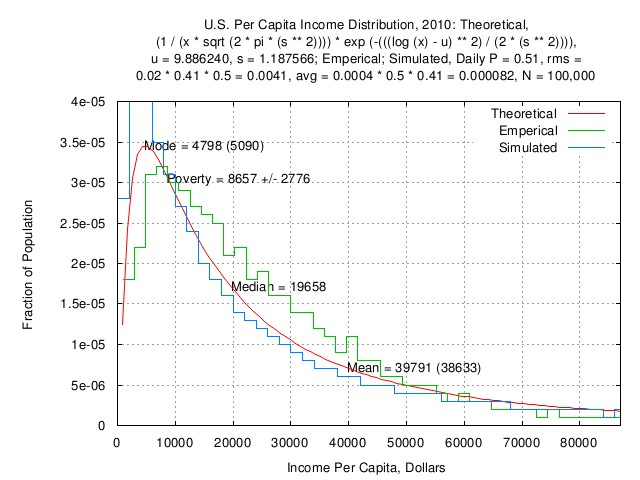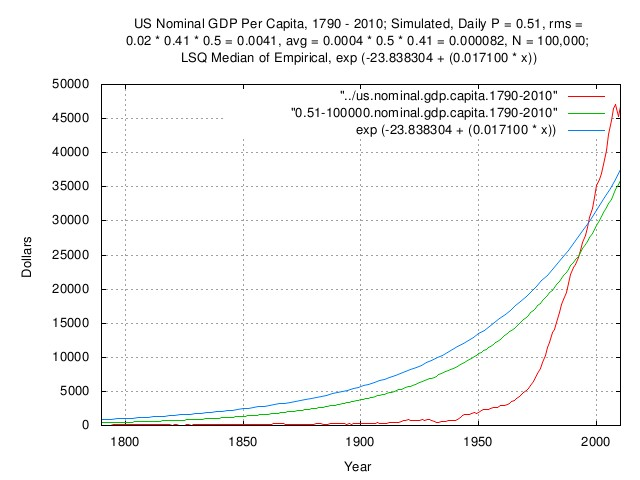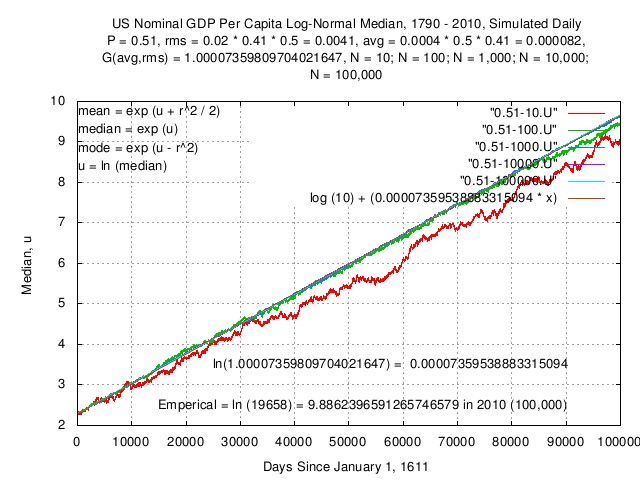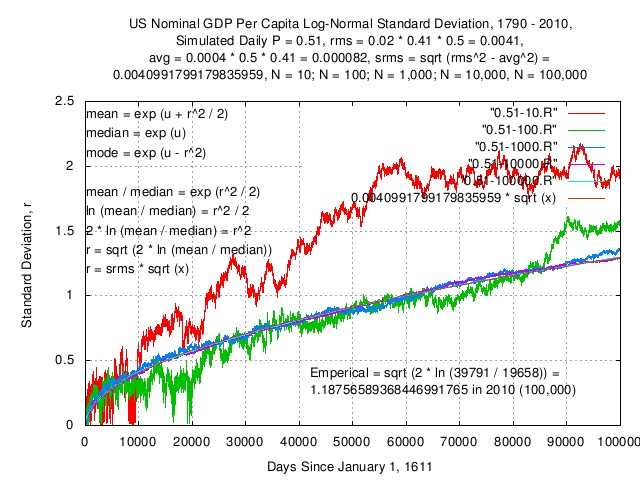john@email.johncon.com http://www.johncon.com/john/

## tsinvestsim-lognormal

### Verification of Analytical Methodology for Evaluating GDP Per Capita:

To demonstrate the validity of the analytical methodology used in the GDP per capita analysis, tsinvestsim(1) simulations were used to evaluate the convergence of the log-normal distribution of GDP per capita with population sizes over five orders of magnitude, (```N = 10``` to ```N = 100,000```,) and each worker in the workforce having `P = 0.51`, ```f = rms = 0.02 * 0.5 * 0.41```, representing a maximally optimal efficiency, (```P = 0.51```, ```f = rms = 0.02```,) with an inefficiency of `0.41` representing the fraction of the population in the workforce, and each worker in the workforce operating with an inefficiency of `0.5`. These variable values are representative for the daily values, per worker, of many industrialized nations.

The output of the tsinvestsim(1) program was summed into an aggregate GDP using the tsinvestsim-lognormal(1) program, (source archive,) to obtain the `mean`, `median`, and `mode` of the simulated GDP, as a time series, with a log-normal distribution, i.e., the GDP per capita distribution.

Since economic data for productivity is available only on a per capita basis, it is not feasible to determine the inefficiencies, (they are lumped together.) The methodology used allows simulations with different variable values for `P`, ```f = rms```, and `avg`. For the current simulations, it is assumed that each worker in the workforce can operate maximally optimal, ```P = 0.51```, and ```f = rms = 0.02```, (```f^2 = rms^2 = avg```, which is maximally optimal, subject to the Kelly Criteria,) and `f = rms` is decreased to represent the inefficiencies. Note that this decreases `avg` by the same inefficiency factor:

``````

P = ((avg / (k * rms)) + 1) / 2
avg = (k * rms) * ((2 * P) - 1)

``````

Where `P` is invariant with `k`, (`avg` is computed by the tsinvestsim(1) program in this manner) Note, further, that:

``````

rms = sqrt (srms^2 + avg^2)
k * rms = k * sqrt (srms^2 + avg^2)
k * rms = sqrt ((k^2 * srms^2) + (k^2 * avg^2))
k * rms = sqrt ((k * srms)^2 + (k * avg)^2)

``````

indicating that `rms` can be multiplied by a constant, `k`, without first calculating `srms` and `avg`, then multiplying each by `k`, squaring each product, and finally taking the root-mean-square of the sum of the squares.

The rationale for selecting variable values in this manner is that `P` is a metric on a worker's ability to synthesize innovation based on new information, (original, secret, or public,) and `f = rms` is a metric on the wager made on the innovation, with `avg` being the return, (positive or negative,) generated by the innovation, i.e., metrics on how smart the worker is, and how good a gambler.

If the national GDP per capita, (an aggregate expenditure approach,) and distribution of income, (an aggregate income approach,) are analyzed together, the variable values for a typical worker in the economy can be determined.

In the following simulations, all workers have the same daily variable values `P = 0.51`, and `f = rms = 0.02 * 0.5 * 0.41`, (for 51 times out of a hundred innovation success rate, 50% efficiency at wagering, and 41% of the population in the workforce,) with each worker starting at ```I = \$10```, (all equal, representing the rural/shared agricultural economy of the US in 1610,) and the simulations are all allowed to run for 100,000 days, (about 4 centuries at 250 work days a year,) developing into a log-normal distribution standard of living, (i.e., GDP growth per capita,) by 1790, and continuing through 2010. The simulation outputs are then sampled every 250 days, for annual data, and all values filtered, prior to 1790, resulting in 1790 through 2010 annual data. The simulation was repeated for workforce sizes of `N = 10`, ```N = 100```, ```N = 1,000```, ```N = 10,000```, and ```N = 100,000```, to verify convergence to the mean of the simulations.

It should be pointed out, as a concluding remark, that the objective of the simulations are validity of methodology which requires only a reasonable approximation to the actual US GDP per capita-and two digit precision variables is adequate.

### Analysis of Simulations:Figure I

Figure I is a plot of the US nominal GDP per capita income distribution, 2010: theoretical distribution, empirical distribution, and, simulated distribution (```N = 100,000```.)Figure 2

Figure 2 is a plot of the US nominal GDP per capita, 1790-2010: empirical values, simulated values (```N = 100,000```,) and Least Squares fit of the empirical values.Figure III

Figure III is a plot of the simulated US GDP per capita log-normal distribution median, over time, with five orders of magnitude of population sizes, `N`, and a plot of the theoretical values for ```N = 100,000```. Note the convergence to the theoretical values with increasing `N`. Further, note that the values converged to are independent of `N`:

``````

P = 0.51
rms = 0.02 * 0.5 * 0.41
avg = (rms) * ((2 * P) - 1)
= 0.000082
G(avg,rms) = 1.00007359809704021647

``````

Or, the value, `V`, at a time `x` many days, starting with an initial value of `I = 10`:

``````

V(x) = I * G(avg,rms)^x

``````

Or:

``````

ln (V(x)) = ln (10) + (x * ln (G(avg,rms)))
= 2.30258509299404568402 + (0.00007359538883315094 * x)

``````

It is important to note that this represents the aggregate growth in the median of the workforce divided by the size of the population, (i.e., the median per capita value,) over time, with the workforce operating at 50% efficiency, (relative to `rms`,) and has a direct mathematical relationship to the typical worker variable values, `rms` and `P`, on a daily basis. This function is exponentiated to obtain the median of the log-normal distribution, over time.Figure IV

Figure IV is a plot of the simulated US nominal GDP per capita log-normal distribution deviation, over time, with five orders of magnitude of population sizes, `N`, and a plot of the theoretical values for `N = 100,000`. Note the convergence to the theoretical values with increasing `N`. Further, note that the values converged to are independent of `N`:

``````

P = 0.51
rms = 0.02 * 0.5 * 0.41
avg = (rms) * ((2 * P) - 1)
= 0.000082
srms = sqrt (rms^2 - avg^2)
= 0.0040991799179835959

``````

Or, the value, `S`, at a time `x` many days:

``````

S(x) = srms * sqrt (x)
= 0.0040991799179835959 * sqrt (x)

``````

It is important to note that this represents the standard deviation of the Gaussian/Normal distribution of the standard of living of the workforce (i.e., the distribution of the per capita values,) over time, with the workforce operating at 50% efficiency, (relative to `rms`,) and has a direct mathematical relationship to the typical worker variable values, `rms` and `P`, on a daily basis. The square of this function, divided by two, is exponentiated to obtain the deviation from the median of the log-normal distribution, over time.

Further, it is important to note that the `srms` of the workers, (or their typical value of the aggregate,) is related to the median and mean of the log-normal distribution at any time.

### Analytical Methodology:

Let `t` be the time interval the log-normal distribution has evolved in the data file, `data.file`, of the GDP per capita. Then, in the `t`'th, (last,) interval:

``````

u = Mu
r = Rho

median = e^u
mean = e^(u + (r^2 / 2))
mode = e^(u - r^2)

``````

If the `data.file` represents GDP per capita data, (i.e., annual GDP divided by annual population count,) then the file represents `mean` GDP per capita data. One of the issues is to convert the data to `median` data.

It is convenient to analyze the evolution of log-normal distribution, meaning by time, `t`:

``````

median(t) = e^u(t)
mean(t) = e^(u(t) + (r(t)^2 / 2))
mode(t) = e^(u(t) - r(t)^2)

``````

where for economic data, (like the US GDP per capita,) the `mean(t)` historical time series and the `median(t)`, `mean(t)`, and `mode(t)` are available only at one point in time, (usually the last interval.)

``````

mean(t) = e^(u(t) + (r(t)^2 / 2))
mode(t) = e^(u(t) - r(t)^2)
mean(t) = e^(u(t)) * e^(r(t)^2 / 2)
mode(t) = e^(u(t)) / e^(r(t)^2)
mean(t) / mode(t) = (e^(u(t)) * e^(r(t)^2 / 2)) / (e^(u(t)) / e^(r(t)^2))
mean(t) / mode(t) = (e^(r(t)^2 / 2)) / (1 / e^(r(t)^2))
mean(t) / mode(t) = (e^(r(t)^2 / 2)) * e^(r(t)^2)
mean(t) / mode(t) = e^((r(t)^2 / 2) + r(t)^2)
mean(t) / mode(t) = e^(r(t)^2 * (1 + (1 / 2)))
mean(t) / mode(t) = e^((3 / 2) * r(t)^2)
ln (mean(t) / mode(t)) = (3 / 2) * r(t)^2
r(t)^2 = (2 / 3) * ln (mean(t) / mode(t))
r(t) = sqrt ((2 / 3) * ln (mean(t) / mode(t)))

``````
``````

r(t) = srms * sqrt (t)
r(t)^2 = srms^2 * t

``````
``````

mean(t) = e^(u(t) + (r(t)^2 / 2))
u(t) = a + (b * t)
mean(t) = e^(a + (b * t) + (srms^2 * t / 2))
mean(t) = e^(a + (b * t) + ((srms^2 / 2) * t))
mean(t) = e^(a + ((b + (srms^2 / 2)) * t))
median(t) = e^u(t) = e^(a + (b * t))

``````

with `a` and `b` determined by LSQ of `mean(t)`.

### Validation:

Unfortunately, the tsinvestsim-lognormal(1) program does not provide the `mode(t)` but as an alternative derivation using the `median(t)` and `mean(t)` for analysis of the simulation:

``````

mean(t) = e^(u(t) + (r(t)^2 / 2))
mean(t) = e^u(t) * e^(r(t)^2 / 2)
median(t) = e^u(t)

mean(t) = e^u(t) * e^(r(t)^2 / 2)
mean(t) / median(t) = e^u(t) * e^(r(t)^2 / 2) / e^u(t)
mean(t) / median(t) = e^(r(t)^2 / 2)
r(t)^2 / 2 = ln (mean(t) / median(t))
r(t)^2 = 2 * ln (mean(t) / median(t))
r(t) = sqrt (2 * ln (mean(t) / median(t)))

r(t) = srms * sqrt (t)
srms = r(t) / sqrt (t)

mean(t) = e^(a + ((b + (srms^2 / 2)) * t))
ln (mean(t)) = a + ((b + (srms^2 / 2)) * t)

``````

The actual values used in the simulation:

``````

P = 0.51, f = 0.02 * 0.50 * 0.41 = 0.0041

f = rms = 0.02 * 0.50 * 0.41 = 0.0041
0.51 = ((avg / 0.0041) + 1) / 2
avg = 0.0041 * ((2 * 0.51) - 1)
= 0.000082
srms = sqrt (rms^2 - avg^2)
= sqrt (0.0041^2 - 0.000082^2)
= 0.0040991799179835959

G(avg,rms) = G(0.0041 * ((2 * 0.51) - 1),0.02 * 0.50 * 0.41)
= 1.00007359809704021647
G(t) = 10 * (1.00007359809704021647^t)
ln (G(t)) = ln (10) + (t * ln (1.00007359809704021647))
= 2.30258509299404568402 + (0.00007359538883315094 * t)

ln (mean(t)) = a + ((b + (srms^2 / 2)) * t)
a = 2.30258509299404568402
b + (srms^2 / 2) = 0.00007359538883315094
b = 0.00007359538883315094 - (srms^2 / 2)
= 0.00007359538883315094 - (0.0040991799179835959^2 / 2)
= 0.00006519375083315094
ln(mean(t)) = 2.30258509299404568402 + (0.00007359538883315094 * t)

``````

And, analyzing the data from the simulations, (the order of the fields from tsinvestsim-lognormal(1) program): "`time`, `minimum`, `median`, `mean`, `maximum`",):

``````

cut -f4 0.51-10 | tslsq -e -p
e^(2.243692 + 0.000091t)
cut -f4 0.51-10 | tsfraction | tsavg -p
0.000089
cut -f4 0.51-10 | tsfraction | tsrms -p
0.002662
G(0.000089,0.002662) = 1.00008546072723226738
ln (G(0.000089,0.002662)) = 0.00008545707567235967
egrep '^99999' 0.51-10
99999       1906.627099     8477.783394     52569.322616    387424.213469
r(100000) = sqrt (2 * ln (52569.322616 / 8477.783394))
= 1.91033175441258050009
srms = 1.91033175441258050009 / sqrt (100000)
= 0.00604099943048917012
r(t) = srms * sqrt (t)
r(t) = 0.00604099943048917012 * sqrt (t)
r(t)^2 / 2 = 0.00001824683705958524 * t
ln (mean(t)) = a + ((b + (srms^2 / 2)) * t)
a = 2.243692
b = 0.000091 - (0.00604099943048917012^2 / 2)
= 0.00007275316294041476
u(t) = 2.243692 + (0.00007275316294041476 * t)

``````
``````

cut -f4 0.51-100 | tslsq -e -p
e^(2.230331 + 0.000083t)
cut -f4 0.51-100 | tsfraction | tsavg -p
0.000084
cut -f4 0.51-100 | tsfraction | tsrms -p
0.000736
G(0.000084,0.000736) = 1.00008373267247867903
ln (G(0.000084,0.000736)) = 0.00008372916709413426
egrep '^99999' 0.51-100
99999       290.545725      12589.060312    43569.000789    1248187.418192
r(100000) = sqrt (2 * ln (43569.000789 / 12589.060312))
= 1.57576501978003208644
srms = 1.57576501978003208644 / sqrt (100000)
= 0.00498300651972517984
r(t) = srms * sqrt (t)
r(t) = 0.00498300651972517984 * sqrt (t)
r(t)^2 / 2 = 0.00001241517698781182 * t
ln (mean(t)) = a + ((b + (srms^2 / 2)) * t)
a = 2.230331
b = 0.000083 - (0.00498300651972517984^2 / 2)
= 0.00007058482301218818
u(t) = 2.230331 + (0.00007058482301218818 * t)

``````
``````

cut -f4 0.51-1000 | tslsq -e -p
e^(2.289082 + 0.000082t)
cut -f4 0.51-1000 | tsfraction | tsavg -p
0.000083
cut -f4 0.51-1000 | tsfraction | tsrms -p
0.000220
G(0.000083,0.000220) = 1.00008297924392550143
ln (G(0.000083,0.000220)) = 0.00008297580133848107
egrep '^99999' 0.51-1000
99999       101.798190      15302.138722    38246.692966    987378.496244
r(100000) = sqrt (2 * ln (38246.692966 / 15302.138722))
= 1.35356159353659762681
srms = 1.35356159353659762681 / sqrt (100000)
= 0.00428033758890269486
r(t) = srms * sqrt (t)
r(t) = 0.00428033758890269486 * sqrt (t)
r(t)^2 / 2 = 0.00000916064493748667 * t
ln (mean(t)) = a + ((b + (srms^2 / 2)) * t)
a = 2.289082
b = 0.000082 - (0.00428033758890269486^2 / 2)
= 0.00007283935506251333
u(t) = 2.289082 + (0.00007283935506251333 * t)

``````
``````

cut -f4 0.51-10000 | tslsq -e -p
e^(2.299558 + 0.000082t)
cut -f4 0.51-10000 | tsfraction | tsavg -p
0.000082
cut -f4 0.51-10000 | tsfraction | tsrms -p
0.000105
G(0.000082,0.000105) = 1.00008199784944121253
ln (G(0.000082,0.000105)) = 0.00008199448780131961
egrep '^99999' 0.51-10000
99999       173.857625      15894.484298    36704.069587    1771254.349566
r(100000) = sqrt (2 * ln (36704.069587 / 15894.484298))
= 1.29376619780877467417
srms = 1.29376619780877467417 / sqrt (100000)
= 0.00409124794481167259
r(t) = srms * sqrt (t)
r(t) = 0.00409124794481167259 * sqrt (t)
r(t)^2 / 2 = 0.00000836915487296287 * t
ln (mean(t)) = a + ((b + (srms^2 / 2)) * t)
a = 2.299558
b = 0.000082 - (0.00409124794481167259^2 / 2)
= 0.00007363084512703713
u(t) = 2.299558 + (0.00007363084512703713 * t)

``````
``````

cut -f4 0.51-100000 | tslsq -e -p
e^(2.300291 + 0.000082t)
cut -f4 0.51-100000 | tsfraction | tsavg -p
0.000082
cut -f4 0.51-100000 | tsfraction | tsrms -p
0.000085
G(0.000082,0.000085) = 1.00008199974949315241
ln(G(0.000082,0.000085)) = 0.00008199638769747028
egrep '^99999' 0.51-100000
99999       39.190364       15804.790282    36694.603142    11503692.146641
r(100000) = sqrt (2 * ln (36694.603142 / 15804.790282))
= 1.29793421590474711612
srms = 1.29793421590474711612 / sqrt (100000)
= 0.00410442837532374379
r(t) = srms * sqrt (t)
r(t) = 0.00410442837532374379 * sqrt (t)
r(t)^2 / 2 = 0.00000842316614408135 * t
ln (mean(t)) = a + ((b + (srms^2 / 2)) * t)
a = 2.300291
b = 0.000082 - (0.00410442837532374379^2 / 2)
= 0.00007357683385591865
u(t) = 2.300291 + (0.00007357683385591865 * t)

``````

Note that:

``````

tsfraction data.file | tsrms -p
rms

``````

is meaningless, (as far as productivity per capita is concerned,) since the natural evolution of the log-normal distribution of the aggregate GDP per capita, will be, given enough time and population, a near perfect exponential where `avg = rms`, and `P = 1`. The `rms` of the workers, (or per capita value of the aggregate,) must be determined from the `mean(t)`, `mode(t)`, and the `median(t)` of the distribution at some time interval, `t`, (usually the last interval.)

Empirical GDP per capita data can present situations where the long term `avg` and `rms` are not nearly equal, which is usually due to governance issues that effect all, (or most,) of the workforce, and `rms` is greater than `avg`. Subtracting, (via root-mean-square,) the typical individual `rms` from the GDP per capita `rms` offers a methodology for analysis of the governance issues, providing the log-normal distribution has evolved for a sufficient time, and the population size is sufficiently large.

### Simulation:

As a concluding note, to align the simulations, (mean-variance simulations of geometric Brownian motion fractals are notoriously unstable,) with the empirical data, 4 data points had to align with the empirical data: the mean of the simulated nominal US GDP per capita with the empirical nominal US GDP per capita at 1790; the simulated nominal US GDP per capita with the empirical nominal US GDP per capita at 2010; and the median; and mean of the log-normal distribution of the productivity/income of the simulated nominal US GDP per capita with the empirical nominal US GDP per capita at 2010. There are 3 variables, which interact: `I`, the starting value for each worker, (`I = \$10`, in 1610, which was used as a scaling factor); `avg`; and, `rms`. Lowering both `avg` and `rms` will decrease the ratio of the mean to the median in 2010, and, increasing `avg`, relative to `rms`, will increase the growth in the nominal US GDP per capita in the simulation. The number of workers, `N`, contributing to the simulated nominal US GDP per capita was increased, in steps of orders of magnitude, until the simulations converged to their mean, indicating sufficient accuracy for comparison with the empirical data. Note that the log-normal distribution income empirical data for 2010 is in 2010 nominal dollars, necessitating an analysis of nominal US GDP per capita, (as opposed to the traditional real GDP per capita.) The empirical log-normal distribution income in 1790 is not available or known, necessitating starting the simulation about two centuries before any data of interest to the analysis to allow a log-normal distribution income to evolve by 1790. The tsinvestsim(1) program from the NtropiX site, (in the tsinvest archive,) was used to generate the individual worker and nominal US GDP per capita time series for the simulation. The simulation for `N = 100,000` took about 20 hours on a 2.5GHz machine.

### calc(1) Macros:

The following calc(1) macros were used for calculation of `P(avg,rms)` and `G(avg,rms)` in ~/.calcrc:

``````

define P (avg, rms) = ((avg / rms) + 1) / 2;
define G (avg, rms) = power (1 + rms, ((avg / rms) + 1) / 2) * \
power (1 - rms, 1 - ((avg / rms) + 1) / 2);

``````

The following calc(1) script is for computing `avg`, `rms`, and, `G(avg,rms)`, given `srms` and `G` obtained empirically from a time series.

``````

#!/usr/local/bin/calc -d -f
#
# A license is hereby granted to reproduce this design for personal,
# non-commercial use.
#
# THIS DESIGN IS PROVIDED "AS IS". THE AUTHOR PROVIDES NO WARRANTIES
# WHATSOEVER, EXPRESSED OR IMPLIED, INCLUDING WARRANTIES OF
# MERCHANTABILITY, TITLE, OR FITNESS FOR ANY PARTICULAR PURPOSE.  THE
# AUTHOR DOES NOT WARRANT THAT USE OF THIS DESIGN DOES NOT INFRINGE
# THE INTELLECTUAL PROPERTY RIGHTS OF ANY THIRD PARTY IN ANY COUNTRY.
#
# So there.
#
#
# Comments and/or problem reports should be addressed to:
#
#     john@email.johncon.com
#
#     http://www.johncon.com/john/
#     http://www.johncon.com/ntropix/
#     http://www.johncon.com/ndustrix/
#     http://www.johncon.com/nformatix/
#     http://www.johncon.com/ndex/
#
# A calc(1) script for binary search-for-solution of: given, srms and
# g; find avg, rms, and, G(avg,rms).
#
# Both the domain and range, between "top" and "bottom" must be
# monotonic increasing on avg; G(avg,rms) is monotonic increasing on
# increasing avg, (starting with avg = rms = 1 to avoid division by
# zero in the calculation of the first iteration of G(avg,rms), and
# start the binary search at avg = 0.5.)
#
# The variables srms and g, are required. The variable g is G(avg,rms)
# to search for, (and must be greater than unity,) given srms, (which
# must be greater than zero):
#
# Real US GDP:
#
srms = 0.02126296324279258349;
g = 1.0170171123188325426;
#
# Nominal US GDP:
#
# srms = 0.02126296324302608945;
# g = 1.02982917935065044642;
#
top = 1;
bottom = 0;
avg = 1;
rms = 1;
temp = 0.0;
#
while (abs ((temp = G(avg,rms)) - g) > 0.0000000000000000001)
{

if (temp < g)
{
bottom = bottom + ((top - bottom) / 2.0);
avg = bottom + ((top - bottom) / 2.0);
rms = sqrt (avg^2 + srms^2);
/* printf ("1: avg = %f, rms = %f, G(avg,rms) = %f\n", avg, rms, temp); */
}

else
{
top = top - ((top - bottom) / 2.0);
avg = top - ((top - bottom) / 2.0);
rms = sqrt (avg^2 + srms^2);
/* printf ("2: avg = %f, rms = %f, G(avg,rms) = %f\n", avg, rms, temp); */
}

}
#
printf ("avg = %f, rms = %f, G(avg,rms) = %f\n", avg, rms, temp);

``````

The information contained herein is private and confidential and dissemination is strictly forbidden, except under the provisions of contractual license.

THE AUTHOR PROVIDES NO WARRANTIES WHATSOEVER, EXPRESSED OR IMPLIED, INCLUDING WARRANTIES OF MERCHANTABILITY, TITLE, OR FITNESS FOR ANY PARTICULAR PURPOSE. THE AUTHOR DOES NOT WARRANT THAT USE OF THIS INFORMATION DOES NOT INFRINGE THE INTELLECTUAL PROPERTY RIGHTS OF ANY THIRD PARTY IN ANY COUNTRY.

So there.

Comments, questions, and problem reports should be addressed to:

john@email.johncon.com

http://www.johncon.com/john/
http://www.johncon.com/ntropix/
http://www.johncon.com/ndustrix/
http://www.johncon.com/nformatix/
http://www.johncon.com/ndex/